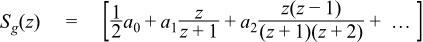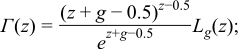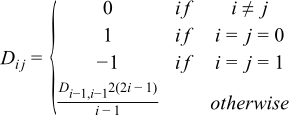#Boost C++ Libraries

...one of the most highly regarded and expertly designed C++ library projects in the world.

This is the documentation for an old version of boost. Click here for the latest Boost documentation.

### The Lanczos Approximation

##### Motivation

Why base gamma and gamma-like functions on the Lanczos approximation?

First of all I should make clear that for the gamma function over real numbers (as opposed to complex ones) the Lanczos approximation (See Wikipedia or Mathworld) appears to offer no clear advantage over more traditional methods such as Stirling's approximation. Pugh carried out an extensive comparison of the various methods available and discovered that they were all very similar in terms of complexity and relative error. However, the Lanczos approximation does have a couple of properties that make it worthy of further consideration:

• The approximation has an easy to compute truncation error that holds for all z > 0. In practice that means we can use the same approximation for all z > 0, and be certain that no matter how large or small z is, the truncation error will at worst be bounded by some finite value.
• The approximation has a form that is particularly amenable to analytic manipulation, in particular ratios of gamma or gamma-like functions are particularly easy to compute without resorting to logarithms.

It is the combination of these two properties that make the approximation attractive: Stirling's approximation is highly accurate for large z, and has some of the same analytic properties as the Lanczos approximation, but can't easily be used across the whole range of z.

As the simplest example, consider the ratio of two gamma functions: one could compute the result via lgamma:

```exp(lgamma(a) - lgamma(b));
```

However, even if lgamma is uniformly accurate to 0.5ulp, the worst case relative error in the above can easily be shown to be:

```Erel > a * log(a)/2 + b * log(b)/2
```

For small a and b that's not a problem, but to put the relationship another way: each time a and b increase in magnitude by a factor of 10, at least one decimal digit of precision will be lost.

In contrast, by analytically combining like power terms in a ratio of Lanczos approximation's, these errors can be virtually eliminated for small a and b, and kept under control for very large (or very small for that matter) a and b. Of course, computing large powers is itself a notoriously hard problem, but even so, analytic combinations of Lanczos approximations can make the difference between obtaining a valid result, or simply garbage. Refer to the implementation notes for the beta function for an example of this method in practice. The incomplete gamma_p gamma and beta functions use similar analytic combinations of power terms, to combine gamma and beta functions divided by large powers into single (simpler) expressions.

##### The Approximation

The Lanczos Approximation to the Gamma Function is given by:Where Sg(z) is an infinite sum, that is convergent for all z > 0, and g is an arbitrary parameter that controls the "shape" of the terms in the sum which is given by:With individual coefficients defined in closed form by:However, evaluation of the sum in that form can lead to numerical instability in the computation of the ratios of rising and falling factorials (effectively we're multiplying by a series of numbers very close to 1, so roundoff errors can accumulate quite rapidly).

The Lanczos approximation is therefore often written in partial fraction form with the leading constants absorbed by the coefficients in the sum:where:Again parameter g is an arbitrarily chosen constant, and N is an arbitrarily chosen number of terms to evaluate in the "Lanczos sum" part.

NoteSome authors choose to define the sum from k=1 to N, and hence end up with N+1 coefficients. This happens to confuse both the following discussion and the code (since C++ deals with half open array ranges, rather than the closed range of the sum). This convention is consistent with Godfrey, but not Pugh, so take care when referring to the literature in this field.
##### Computing the Coefficients

The coefficients C0..CN-1 need to be computed from N and g at high precision, and then stored as part of the program. Calculation of the coefficients is performed via the method of Godfrey; let the constants be contained in a column vector P, then:

P = B D C F

where B is an NxN matrix:D is an NxN matrix:C is an NxN matrix:and F is an N element column vector:Note than the matrices B, D and C contain all integer terms and depend only on N, this product should be computed first, and then multiplied by F as the last step.

##### Choosing the Right Parameters

The trick is to choose N and g to give the desired level of accuracy: choosing a small value for g leads to a strictly convergent series, but one which converges only slowly. Choosing a larger value of g causes the terms in the series to be large and/or divergent for about the first g-1 terms, and to then suddenly converge with a "crunch".

Pugh has determined the optimal value of g for N in the range 1 <= N <= 60: unfortunately in practice choosing these values leads to cancellation errors in the Lanczos sum as the largest term in the (alternating) series is approximately 1000 times larger than the result. These optimal values appear not to be useful in practice unless the evaluation can be done with a number of guard digits and the coefficients are stored at higher precision than that desired in the result. These values are best reserved for say, computing to float precision with double precision arithmetic.

Table 57. Optimal choices for N and g when computing with guard digits (source: Pugh)

Significand Size

N

g

Max Error

24

6

5.581

9.51e-12

53

13

13.144565

9.2213e-23

The alternative described by Godfrey is to perform an exhaustive search of the N and g parameter space to determine the optimal combination for a given p digit floating-point type. Repeating this work found a good approximation for double precision arithmetic (close to the one Godfrey found), but failed to find really good approximations for 80 or 128-bit long doubles. Further it was observed that the approximations obtained tended to optimised for the small values of z (1 < z < 200) used to test the implementation against the factorials. Computing ratios of gamma functions with large arguments were observed to suffer from error resulting from the truncation of the Lancozos series.

Pugh identified all the locations where the theoretical error of the approximation were at a minimum, but unfortunately has published only the largest of these minima. However, he makes the observation that the minima coincide closely with the location where the first neglected term (aN) in the Lanczos series Sg(z) changes sign. These locations are quite easy to locate, albeit with considerable computer time. These "sweet spots" need only be computed once, tabulated, and then searched when required for an approximation that delivers the required precision for some fixed precision type.

Unfortunately, following this path failed to find a really good approximation for 128-bit long doubles, and those found for 64 and 80-bit reals required an excessive number of terms. There are two competing issues here: high precision requires a large value of g, but avoiding cancellation errors in the evaluation requires a small g.

At this point note that the Lanczos sum can be converted into rational form (a ratio of two polynomials, obtained from the partial-fraction form using polynomial arithmetic), and doing so changes the coefficients so that they are all positive. That means that the sum in rational form can be evaluated without cancellation error, albeit with double the number of coefficients for a given N. Repeating the search of the "sweet spots", this time evaluating the Lanczos sum in rational form, and testing only those "sweet spots" whose theoretical error is less than the machine epsilon for the type being tested, yielded good approximations for all the types tested. The optimal values found were quite close to the best cases reported by Pugh (just slightly larger N and slightly smaller g for a given precision than Pugh reports), and even though converting to rational form doubles the number of stored coefficients, it should be noted that half of them are integers (and therefore require less storage space) and the approximations require a smaller N than would otherwise be required, so fewer floating point operations may be required overall.

The following table shows the optimal values for N and g when computing at fixed precision. These should be taken as work in progress: there are no values for 106-bit significand machines (Darwin long doubles & NTL quad_float), and further optimisation of the values of g may be possible. Errors given in the table are estimates of the error due to truncation of the Lanczos infinite series to N terms. They are calculated from the sum of the first five neglected terms - and are known to be rather pessimistic estimates - although it is noticeable that the best combinations of N and g occurred when the estimated truncation error almost exactly matches the machine epsilon for the type in question.

Table 58. Optimum value for N and g when computing at fixed precision

Significand Size

Platform/Compiler Used

N

g

Max Truncation Error

24

Win32, VC++ 7.1

6

1.428456135094165802001953125

9.41e-007

53

Win32, VC++ 7.1

13

6.024680040776729583740234375

3.23e-016

64

Suse Linux 9 IA64, gcc-3.3.3

17

12.2252227365970611572265625

2.34e-024

116

HP Tru64 Unix 5.1B / Alpha, Compaq C++ V7.1-006

24

20.3209821879863739013671875

4.75e-035

Finally note that the Lanczos approximation can be written as follows by removing a factor of exp(g) from the denominator, and then dividing all the coefficients by exp(g):This form is more convenient for calculating lgamma, but for the gamma function the division by e turns a possibly exact quality into an inexact value: this reduces accuracy in the common case that the input is exact, and so isn't used for the gamma function.

##### References
1. Paul Godfrey, "A note on the computation of the convergent Lanczos complex Gamma approximation".
2. Glendon Ralph Pugh, "An Analysis of the Lanczos Gamma Approximation", PhD Thesis November 2004.
3. Viktor T. Toth, "Calculators and the Gamma Function".
4. Mathworld, The Lanczos Approximation.
 Copyright © 2006-2010 John Maddock, Paul A. Bristow, Hubert Holin, Xiaogang Zhang, Bruno Lalande, Johan Råde, Gautam Sewani and Thijs van den Berg Distributed under the Boost Software License, Version 1.0. (See accompanying file LICENSE_1_0.txt or copy at http://www.boost.org/LICENSE_1_0.txt)